## Interns Explain Basic Neural Network

AI Insights

Neural Network is an algorithm that is inspired by the neurons in the brain. Each unit computes the output using an “activation function” and send the layer activation to be an input for the units in the next layer.

#### Activation Functions

An activation function is a function used to compute the output in a layer of a Neural Network. These are examples of activation functions that are commonly used in neural networks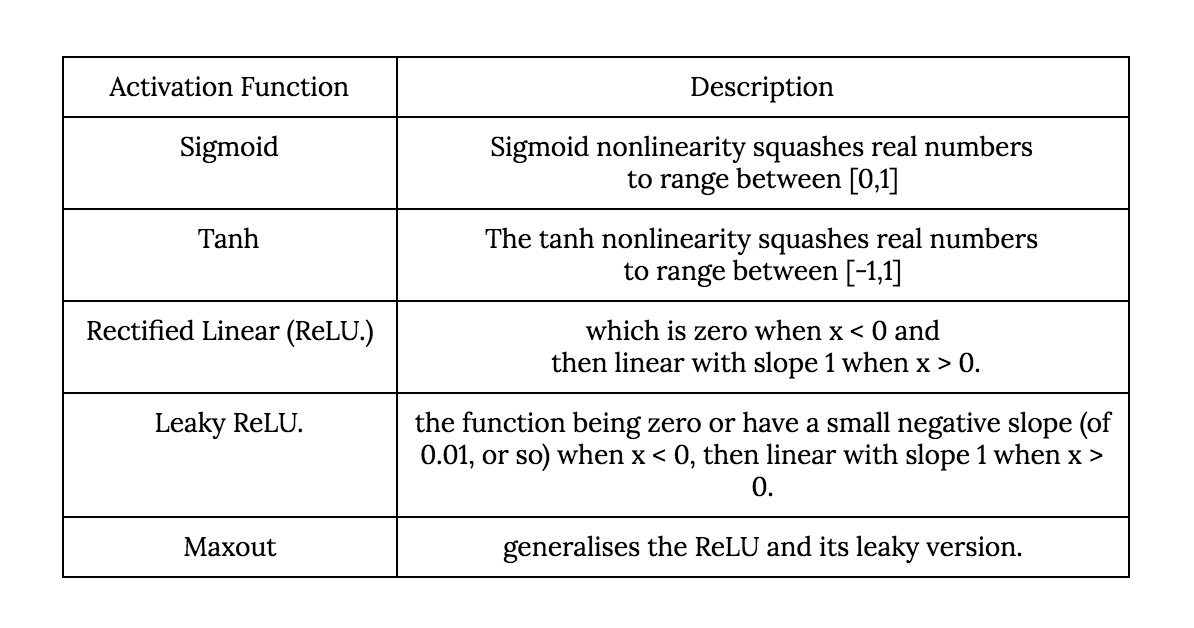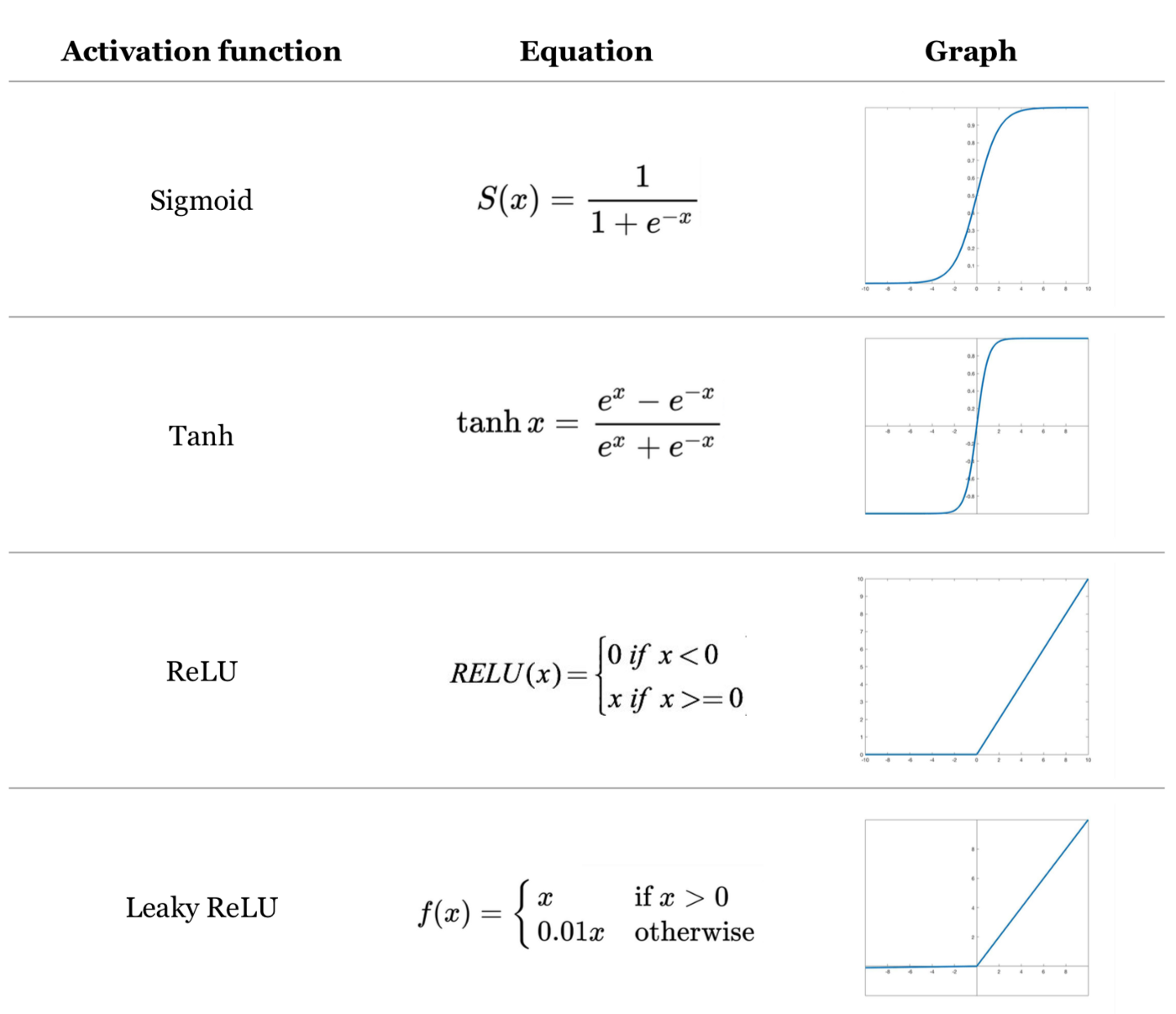#### Cost Function

A cost function is a function that measures the accuracy of our hypothesis function by the difference between the predicted value and the actual value.

Different hypotheses may use different cost functions. A common cost function used in neural networks is the categorical cross-entropy with regularization: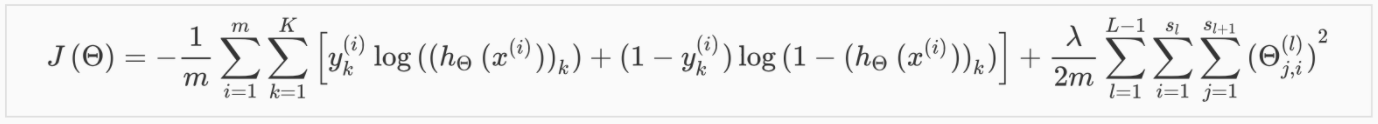L = total number of layers in the network

S_l = number of units (not counting bias unit) in layer l

K = number of output units or number of classes

#### Forward Propagation & Back Propagation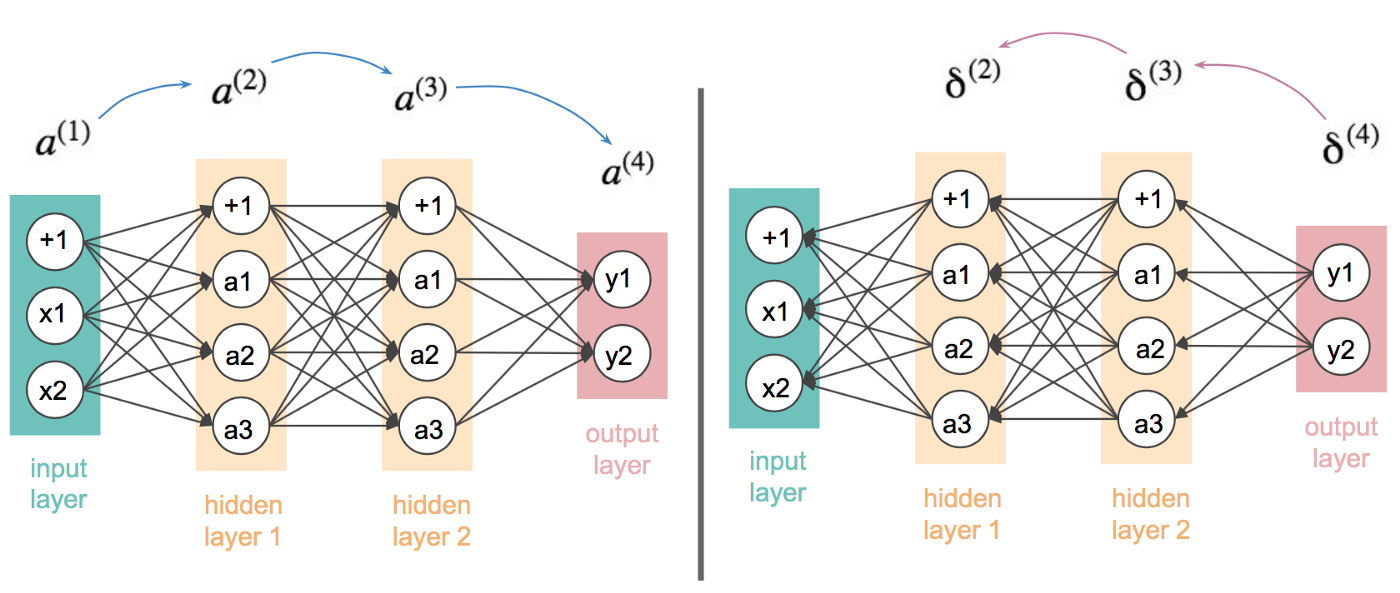After randomly initializing all weights and parameters in the network, the network will start to compute outputs by calculating the weighted sum of each neuron from the previous layer, then using activation function that you defined, compute the output into the next layer until we reach the last layer, thus getting our target prediction. This process is called “forward propagation”. Of course because with random initialization, the network will certainly make a mistake for the prediction. Whatever our prediction is, the network will compute the error from the true value, and propagate the error signal back through the entire network. This process is called “back propagation”. The process is repeated until the cost is minimized to a certain point to which we call convergence.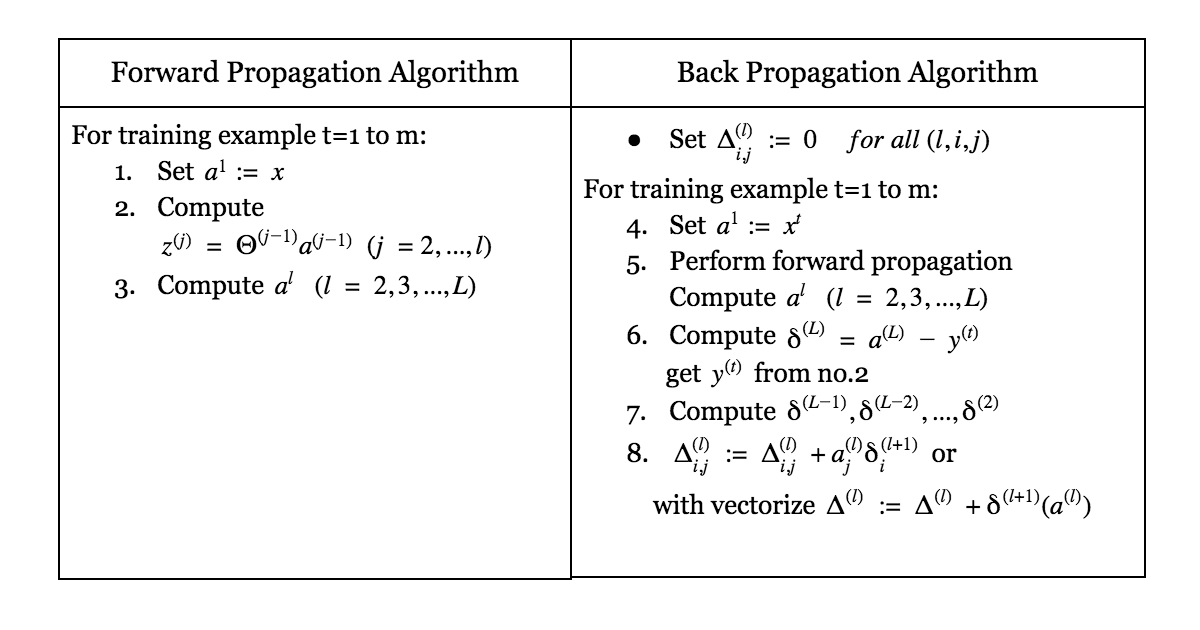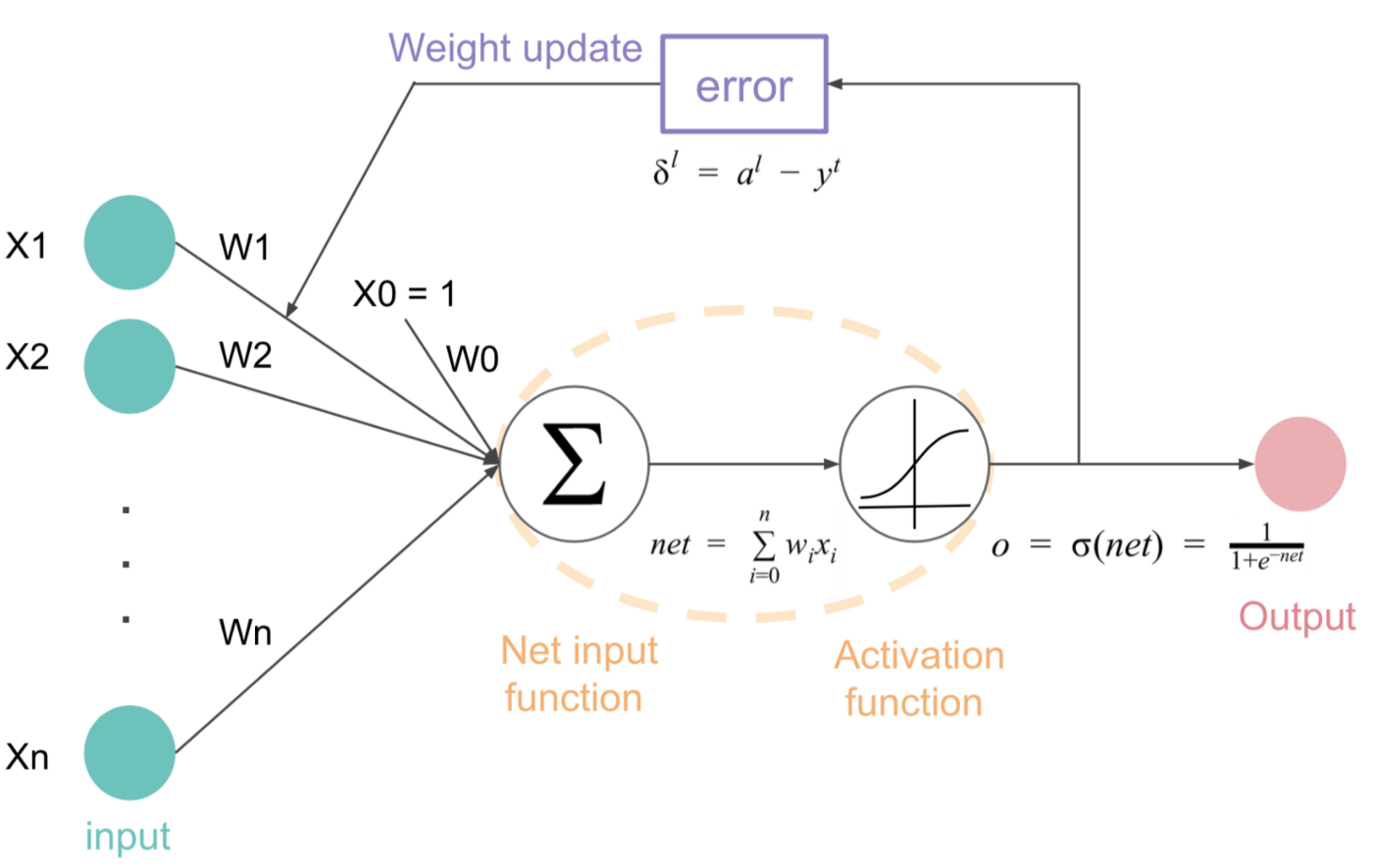A neural network with 1 activation unit. The activation unit summarizes all inputs including bias unit (x0). Then compute output using activation function. After that, the network will compute cost function and sent the error back to adjust weights until we get minimize cost.

Gradient Descent is one of the optimization techniques commonly used in machine learning.

In neural networks, the gradient descent algorithm is used for finding a local minimum of a cost function.

The algorithm is: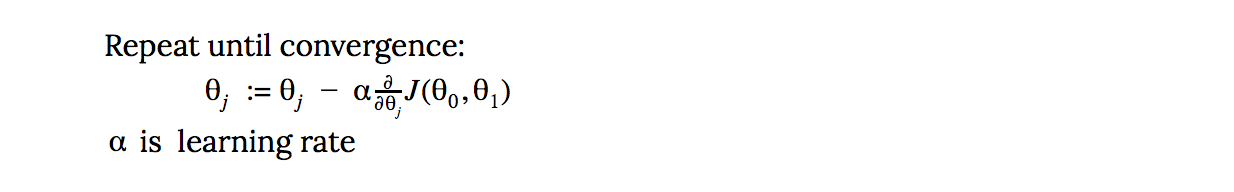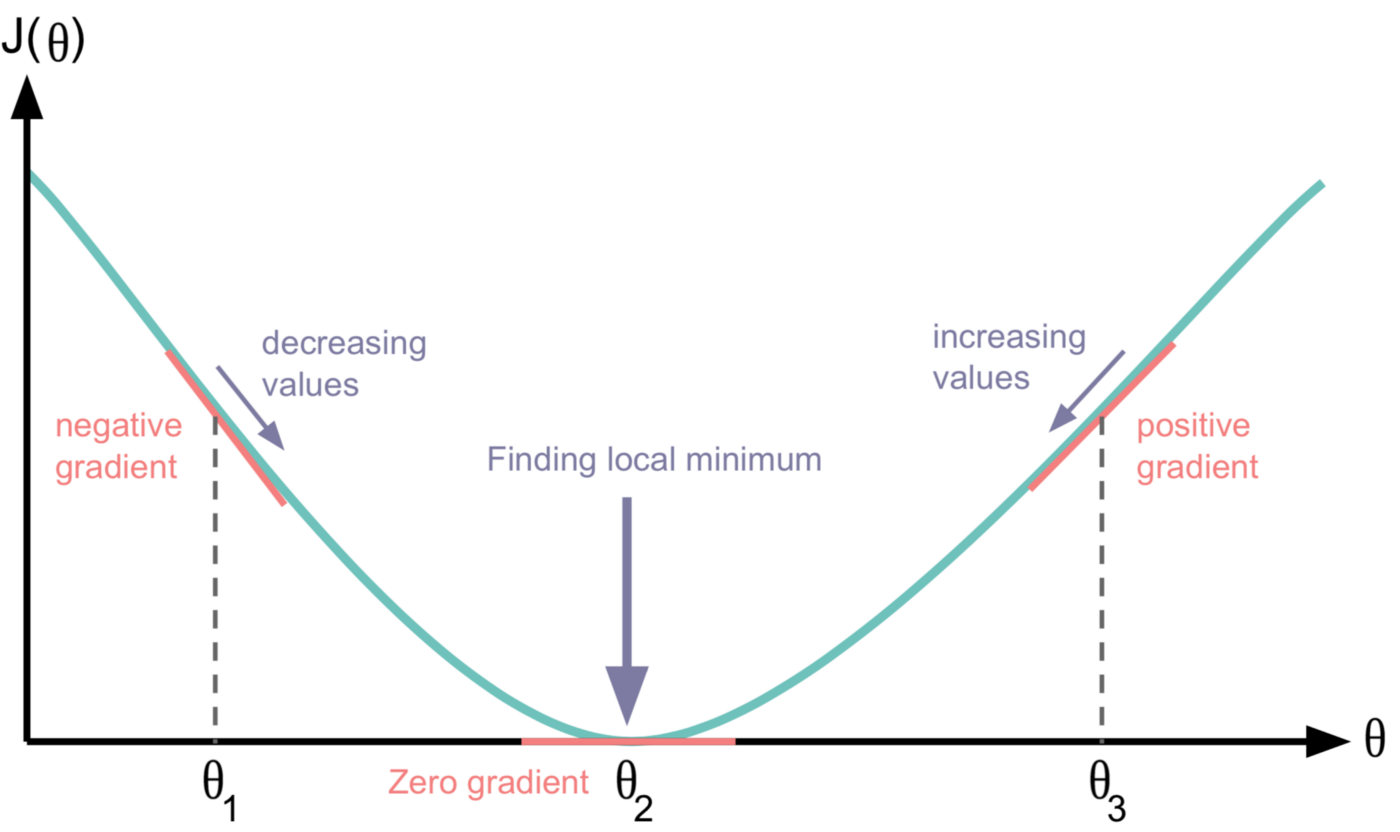To find the local minimum. Let see the picture above

• What direction is defined by the negative slope of the graph• How big of a step is defined by the learning rate (here we call it alpha)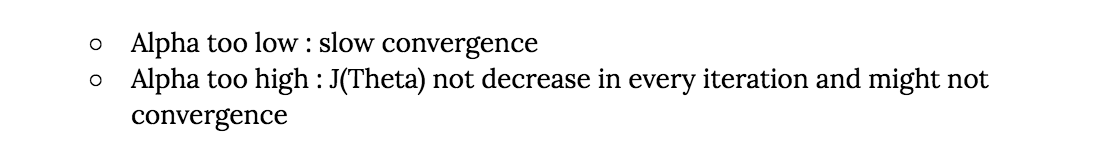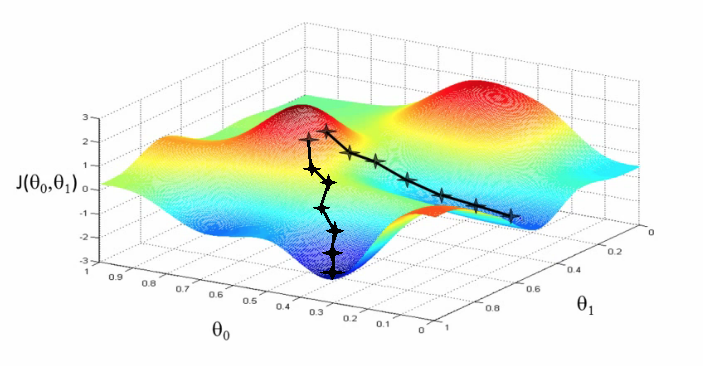Remark: Gradient Descent guarantees only finding a “local minimum”, not the “global minimum”. So if we start at a different position (initialize different values of parameters), we might end up getting a different minimum cost function and a different set of weights and parameters.